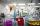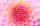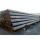Evaluation of expressions

If a2-3a+1=0, find

(i)a2+1/a2
(ii) a3+1/a3

Result

x1 =  7
x2 =  7
y1 =  18
y2 =  18

Solution:Checkout calculation with our calculator of quadratic equations.Leave us a comment of this math problem and its solution (i.e. if it is still somewhat unclear...):Be the first to comment!To solve this verbal math problem are needed these knowledge from mathematics:

Looking for help with calculating roots of a quadratic equation? Do you have a linear equation or system of equations and looking for its solution? Or do you have quadratic equation?

Next similar math problems:

1. Expressions 3If k(x+6)= 4x2 + 20, what is k(10)=?
2. AlgebraX+y=5, find xy (find the product of x and y if x+y = 5)
3. Expression with powersIf x-1/x=5, find the value of x4+1/x4Find the roots of the quadratic equation: 3x2-4x + (-4) = 0.
5. Square root 2If the square root of 3m2 +22 and -x = 0, and x=7, what is m?
6. Solve equationsolve equation: ?
7. Difference AP 4Calculate the difference of the AP if a1 = 0.5, a2 + a3 = -1.1
8. Crystal waterThe chemist wanted to check the content of water of crystallization of chromic potassium alum K2SO4 * Cr2 (SO4) 3 * 24 H2O, which was a long time in the laboratory. From 96.8 g of K2SO4 * Cr2 (SO4) 3 * 24 H2O prepared 979 cm3 solution of base. S
9. RootsDetermine the quadratic equation absolute coefficient q, that the equation has a real double root and the root x calculate: ?
10. DiscriminantDetermine the discriminant of the equation: ?
11. EquationEquation ? has one root x1 = 8. Determine the coefficient b and the second root x2.
12. Solve 3Solve quadratic equation: (6n+1) (4n-1) = 3n2
13. Reciprocal equation 2Solve this equation: x + 5/x - 6 = 4/11
14. Variation equationSolve combinatorics equation: V(2, x+8)=72
15. TubesIron tubes in the warehouse are stored in layers so that each tube top layer fit into the gaps of the lower layer. How many layers are needed to deposit 100 tubes if top layer has 9 tubes? How many tubes are in bottom layer of tubes?Quadratic equation ? has roots x1 = -26 and x2 = -86. Calculate the coefficients b and c.We want to prove the sentence: If the natural number n is divisible by six, then n is divisible by three. From what assumption we started?